Finding Standard Deviation "By-Hand"
Using the lists, the calculator can simulate a spreadsheet style
"by hand" computation of standard deviation.
(see Measures of Dispersion for the FAST way to find standard deviation)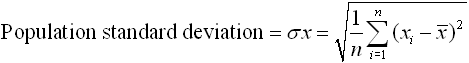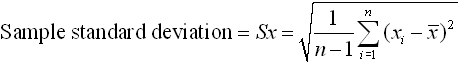Problem:  For the data set {10, 12, 40, 35, 14, 24, 13, 21, 42, 30}, find the range, the standard deviation, the variance, and the mean absolute deviation to the nearest hundredth.

 1.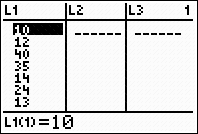Enter the data in L1. 2.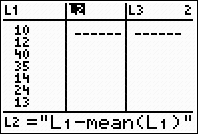Create L2 to be the deviations,. 2nd LIST → MATH #3 mean Using the quotes (ALPHA +) will create a dynamic situation where L2 will automatically update when L1 changes. 3.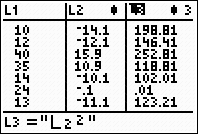Create L3 to be the deviations squared. Again, using the quotes (ALPHA +) will create a dynamic situation where L3 will automatically update when L2 changes. 4.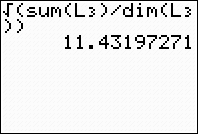From the HOME screen. Compute the sum of the squared deviations, divide by the number of pieces of data (for a population form), and take the square root. 2nd LIST → MATH #5 sum 2nd LIST → OPS #3 dim gives the dimension (size) of the list. ANSWER: population standard deviation is 11.43